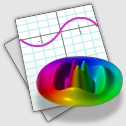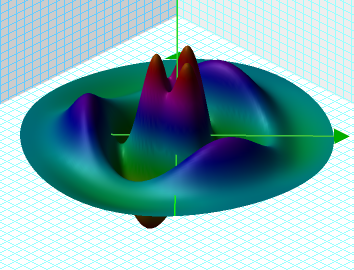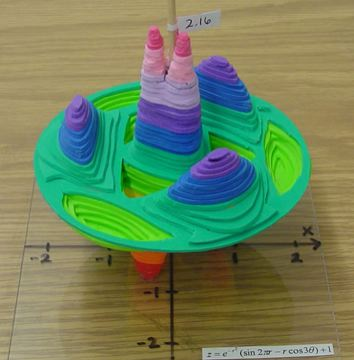### 3D Models

Now these are real 3D models (2004, 2005, 2006 3D projects) .The equation for the surface on the Mac OS X Graphing Calculator application icon above is shown here. Graphing Calculator draws the equation in the image below.Here the equation is modelled in what looks like plasticine.